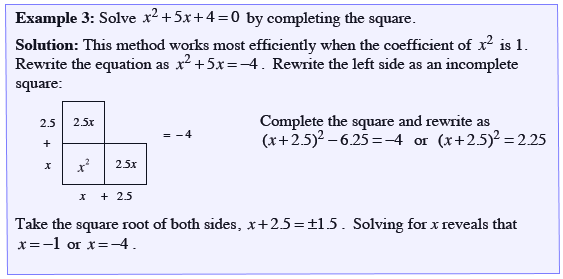### Home > INT2 > Chapter 12 > Lesson 12.1.2 > Problem12-24

12-24.

For the quadratic function $f(x) = x^2 + 6x + 11$: Homework Help ✎

1. Complete the square to write the equation in graphing form.

Use the Math Notes box.2. State the vertex and sketch a graph of the parabola.

In the standard equation, the vertex is $(h, k)$.

$(-3, 2)$

3. What does the graph tell you about the solutions to the equation $x^2 + 6x + 11 = 0$? Explain.

Are there any $x$-intercepts?